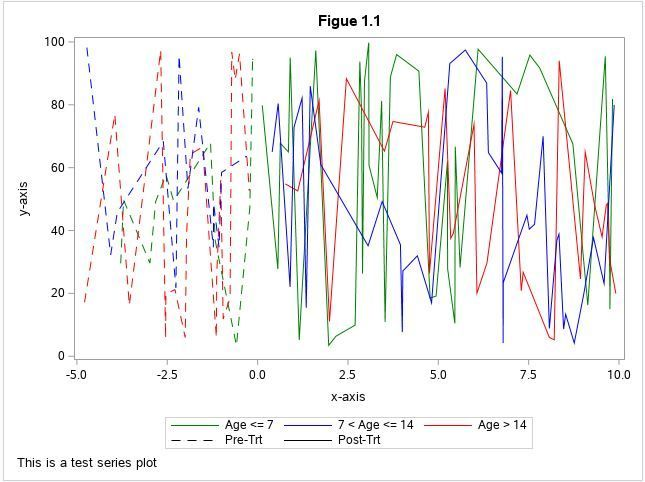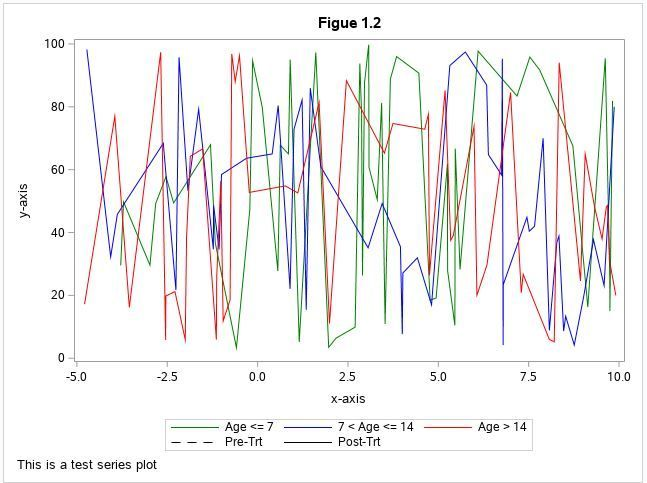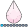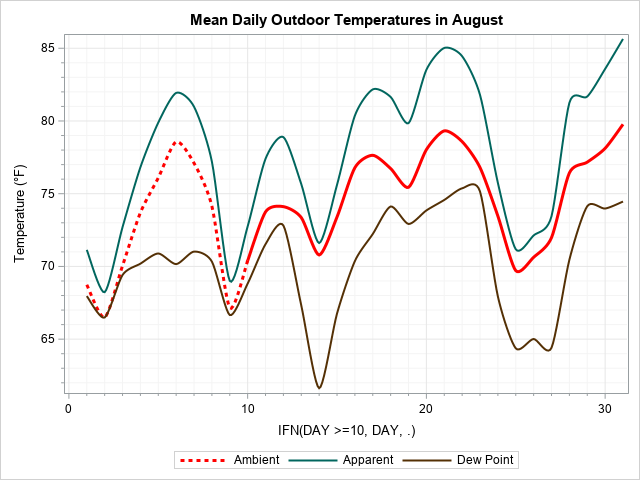## SGPLOT - how to find a way to create series plot with line attributes dependent from two factors

Hi,

I am trying to create series plot using SGPLOT. The assumptions are that lines should be dash when result is collected before starting point (0 on x-axis) and solid when result is collected after starting point. I should draw as many lines as subjects in the input dataset and these lines should be colored depending of subject age (3 groups - 3 colors). I used two approaches but not get 100% what I want.

The first one is to call series statement two times - one for data pre and second for data post. Then use grouplc for assigning correct colors. It works almost fine but there is a break between dashed line and solid line when plot passes 0 point. The code which I am using is:

``````title "Figue 1.1";
footnote1 j=l "This is a test series plot";

proc sgplot data=plot_data3 dattrmap=myattrmap;
series x=x_pre y=y_pre / name="plot1" group=subj groupdisplay=overlay grouplc=fl_a lcattrid=myid2 lineattrs=(pattern=dash);
series x=x_post y=y_post / name="plot2" group=subj groupdisplay=overlay grouplc=fl_a lcattrid=myid2 lineattrs=(pattern=solid);

xaxis label="x-axis";
yaxis label="y-axis";
legenditem type=line name="dash_line" / lineattrs=(pattern=dash color=black thickness=1.0pt) label="Pre-Trt";
legenditem type=line name="solid_line" / lineattrs=(pattern=solid color=black thickness=1.0pt) label="Post-Trt";
legenditem type=line name="age1" / lineattrs=(pattern=solid color=green thickness=1.0pt) label="Age <= 7";
legenditem type=line name="age2" / lineattrs=(pattern=solid color=blue thickness=1.0pt) label="7 < Age <= 14";
legenditem type=line name="age3" / lineattrs=(pattern=solid color=red thickness=1.0pt) label="Age > 14";

keylegend "age1" "age2" "age3" "dash_line" "solid_line" ;
run;``````

Result:The second approach is to call series three times - one for each age group and use grouplp= but it is not working. I get only solid lines with this.

``````title "Figue 1.2";
footnote1 j=l "This is a test series plot";

proc sgplot data=plot_data3 dattrmap=myattrmap;
series x=x_g y=y_g / name="plot1" group=subj groupdisplay=overlay grouplp=fl_p lpattrid=myid1 lineattrs=(color=green); /* grouplp not working */
series x=x_b y=y_b / name="plot2" group=subj groupdisplay=overlay grouplp=fl_p lpattrid=myid1 lineattrs=(color=blue); /* grouplp not working */
series x=x_r y=y_r / name="plot3" group=subj groupdisplay=overlay grouplp=fl_p lpattrid=myid1 lineattrs=(color=red); /* grouplp not working */

xaxis label="x-axis";
yaxis label="y-axis";
legenditem type=line name="dash_line" / lineattrs=(pattern=dash color=black thickness=1.0pt) label="Pre-Trt";
legenditem type=line name="solid_line" / lineattrs=(pattern=solid color=black thickness=1.0pt) label="Post-Trt";
legenditem type=line name="age1" / lineattrs=(pattern=solid color=green thickness=1.0pt) label="Age <= 7";
legenditem type=line name="age2" / lineattrs=(pattern=solid color=blue thickness=1.0pt) label="7 < Age <= 14";
legenditem type=line name="age3" / lineattrs=(pattern=solid color=red thickness=1.0pt) label="Age > 14";

keylegend "age1" "age2" "age3" "dash_line" "solid_line";
run;``````

Result:I can "cheat" in case of first option and prepare data that last row of pre group is the same as the first row of the post group but doing this can lead to situation that I have solid line before 0 point on x-axis and it will be confusing. Is there any better solution for this problem, please?

Below the rest of the code (data preparation).

``````title; footnote;

data plot_data;
do i=1 to 3;
subj="S"||strip(put(i,z3.));
if i=1 then age=6;
if i=2 then age=12;
if i=3 then age=18;
age=22*ranuni(22)+3;
do j=1 to 50;
x=15*ranuni(22)-5;  /* (b,a+b) */
y=99*ranuni(22)+1;
output;
end;
end;

attrib x label="AYEAR"
y label="REUSLT";

drop i j;
run;

data plot_data2;
set plot_data;
length fl_p \$5. fl_a \$10.;

if x < 0 then fl_p="PRE";
if x > 0 then fl_p="POST";

if age < 7 then fl_a="GREEN";
else if 7 <= age < 14 then fl_a="BLUE";
else if age > 14 then fl_a="RED";

fl_all=compress(cat(fl_p,"_", fl_a));
run;

data plot_data3;
set plot_data2;

if fl_p="PRE" then do;
x_pre=x; y_pre=y;
end;

if fl_p="POST" then do;
x_post=x; y_post=y;
end;

if fl_a="GREEN" then do;
x_g=x; y_g=y;
end;

if fl_a="BLUE" then do;
x_b=x; y_b=y;
end;

if fl_a="RED" then do;
x_r=x; y_r=y;
end;
run;

proc sort data=plot_data3;
by subj x;
run;

data myattrmap;
length id \$ 10 value \$ 10 linecolor \$ 9 linepattern \$ 9;
input ID \$ value \$ linecolor \$ linepattern \$;
datalines;
myid1 PRE black dash
myid1 POST black solid
myid2 GREEN green solid
myid2 BLUE blue solid
myid2 RED red solid
;
run;``````

Best Regards!

3 REPLIES 3djrisks
Barite | Level 11

## Re: SGPLOT - how to find a way to create series plot with line attributes dependent from two factors

Hi @Klosiak

Here's an example where I got different line patterns before a cut off. Look at the red curve. Although, I did find it strange that I had to use <=&cutoff, and >=&cutoff so that there is no break in between. Therefore I think if you just have two columns of data, i.e. x and y and use this code directly below, instead of your SERIES statements, then it should work with you too.

series x=eval(ifn(x<=0, x, .)) y=y / name="plot1" group=subj groupdisplay=overlay grouplc=fl_a lcattrid=myid2 lineattrs=(pattern=dash);
series x=(ifn(x>=0, y, .)) y=y / name="plot2" group=subj groupdisplay=overlay grouplc=fl_a lcattrid=myid2 lineattrs=(pattern=solid);

``````/* Mean temperature data for August in 2014. */
data augusttemps;
input day ambient dewpoint apparent;
label day="Day of Month" Ambient="Ambient"
Apparent="Apparent" Dewpoint="Dew Point";
datalines;
1   68.74  67.96  71.14
2   66.49  66.49  68.23
3   70.05  69.41  72.70
4   73.70  70.19  76.82
5   76.09  70.89  79.89
6   78.57  70.16  81.93
7   77.10  71.02  80.98
8   74.14  70.33  77.20
9   67.16  66.66  69.01
10  70.39  68.82  72.75
11  73.75  71.53  77.40
12  74.11  72.81  78.89
13  73.36  67.32  75.66
14  70.79  61.63  71.61
15  73.40  66.76  75.61
16  76.79  70.37  80.37
17  77.64  72.23  82.17
18  76.73  74.12  81.65
19  75.44  72.92  79.85
20  78.03  73.85  83.52
21  79.32  74.57  85.02
22  78.58  75.37  84.45
23  76.80  75.15  81.79
24  73.46  67.93  75.74
25  69.71  64.37  71.18
26  70.63  65.01  72.13
27  71.98  64.41  73.45
28  76.43  70.46  81.25
29  77.17  74.14  81.68
30  78.12  73.98  83.58
31  79.77  74.46  85.64
;
run;

data augusttemps2;
set augusttemps;
if day <= 20 then group = 1;
else group = 2;
run;

/* Define the graph template */
proc template;
define statgraph seriesoverlay;
begingraph;
entrytitle "Mean Daily Outdoor Temperatures in August";
layout overlay / cycleattrs=true
xaxisopts=(type=linear griddisplay=on
linearopts=(minorticks=true minortickcount=9
minorgrid=true))
yaxisopts=(type=linear
label="Temperature ((*ESC*){unicode '00B0'x}F)"
griddisplay=on
linearopts=(minorticks=true minortickcount=4
minorgrid=true));

* Difference in line patterns;
seriesplot x= eval(ifn(day >=10, day, .)) y=ambient / name="ambient"
smoothconnect=true lineattrs=(thickness=3px color=red);
seriesplot x= eval(ifn(day <=10, day, .)) y=ambient / name="ambient"
smoothconnect=true lineattrs=(thickness=3px pattern=2 color=red);

seriesplot x=day y=apparent / name="apparent"
smoothconnect=true
lineattrs=(thickness=2px);
seriesplot x=day y=dewpoint / name="dewpoint"
smoothconnect=true
lineattrs=(thickness=2px);
discretelegend "ambient" "apparent" "dewpoint";
endlayout;
endgraph;
end;
run;

/* Render the graph */
proc sgrender data=augusttemps2 template=seriesoverlay;
run;``````djrisks
Barite | Level 11

## Re: SGPLOT - how to find a way to create series plot with line attributes dependent from two factors## Re: SGPLOT - how to find a way to create series plot with line attributes dependent from two factors

Hi @djrisks

Thanks for sharing this example with me. I can confirm that it works fine when used in proc template (expressions are allowed there). It won't work inside proc sgplot as expressions are not available in series command within this procedure.

Unfortunately this approach is not solving my issue. It will work when both parts of data are linked by one row where the cut-off goes.

Please have a look at this example set of data which I am dealing with:

``````data example01;
infile datalines delimiter=',';
input id \$ x y;
datalines;
1111111,-2.72689938398357,-20.7865168539325
1111111,-2.49144421629021,-13.4831460674157
1111111,-2.00958247775496,-21.9101123595505
1111111,-1.49486652977412,-16.5730337078651
1111111,-1.01300479123887,-5.89887640449438
1111111,-0.00273785078713,0
1111111,0.2819986310746,-12.3595505617977
1111111,0.60780287474332,-16.0112359550561
1111111,0.93634496919917,-20.2247191011235
1111111,1.24024640657084,-21.067415730337
1111111,1.5523613963039,-23.314606741573
1111111,1.8425735797399,-20.7865168539325
1111111,2.33264887063655,-20.2247191011235
1111111, 2.77891854893908,-20.2247191011235
;
run;``````

For x >= 0 I should draw solid line and for x < 0 dash line but as you can see "0" is not a cutoff point. Is there any other approach to solve this besides adding last row before cutoff data as a first row of the post cutoff data?

Best Regards,

Michał

Discussion stats
• 3 replies
• 678 views
• 0 likes
• 2 in conversation